# Long Division with Remainders

When we are given a long division to do it will not always work out to a whole number.

Sometimes there are numbers left over. These are called remainders.

Taking an example similar to that on the Long Division page it becomes more clear:(If you feel happy with the process on the Long Division page you can skip the first bit.)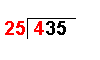4 ÷ 25 = 0 remainder 4 The first number of the dividend is divided by the divisor.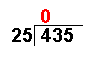The whole number result is placed at the top. Any remainders are ignored at this point.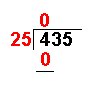25 × 0 = 0 The answer from the first operation is multiplied by the divisor. The result is placed under the number divided into.4 − 0 = 4 Now we take away the bottom number from the top number.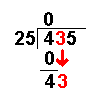Bring down the next number of the dividend.43 ÷ 25 = 1 remainder 18 Divide this number by the divisor.The whole number result is placed at the top. Any remainders are ignored at this point.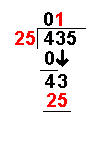25 × 1 = 25 The answer from the above operation is multiplied by the divisor. The result is placed under the last number divided into.43 − 25 = 18 Now we take away the bottom number from the top number.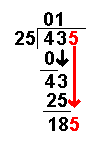Bring down the next number of the dividend.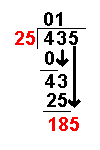185 ÷ 25 = 7 remainder 10 Divide this number by the divisor.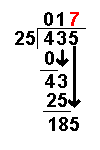The whole number result is placed at the top. Any remainders are ignored at this point.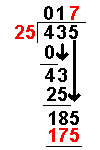25 × 7 = 175 The answer from the above operation is multiplied by the divisor. The result is placed under the number divided into.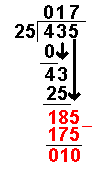185 − 175 = 10 Now we take away the bottom number from the top number. There is still 10 left over but no more numbers to bring down.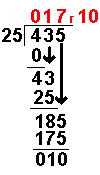With a long division with remainders the answer is expressed as 17 remainder 10 as shown in the diagram   Answer: 435 ÷ 25 = 17 R 10

1651, 1652, 1653, 1654, 1655, 1656, 3437, 3438, 3439, 3440